# CBSE Class 10 Science Electricity Assignment Set A

Read and download free pdf of CBSE Class 10 Science Electricity Assignment Set A. Get printable school Assignments for Class 10 Science. Standard 10 students should practise questions and answers given here for Chapter 12 Electricity Science in Grade 10 which will help them to strengthen their understanding of all important topics. Students should also download free pdf of Printable Worksheets for Class 10 Science prepared as per the latest books and syllabus issued by NCERT, CBSE, KVS and do problems daily to score better marks in tests and examinations

## Assignment for Class 10 Science Chapter 12 Electricity

Class 10 Science students should refer to the following printable assignment in Pdf for Chapter 12 Electricity in standard 10. This test paper with questions and answers for Grade 10 Science will be very useful for exams and help you to score good marks

### Chapter 12 Electricity Class 10 Science Assignment

Question. The rest positions of the needles in a millimeter and voltmeter not in use are as shown in Fig a. when a student used these in his experiment, the readings of the needle are in the positions shown in Fig. B. The corrected values of current and voltage in the experiment are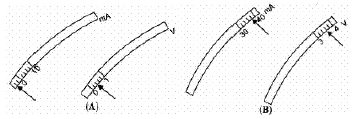a. 34 mA and 4.0 V
b. 42 mA and 4.0 V
c. 34 mA and 3.2 V
d. 42 mA and 3.2 V
Explanation: The miliammeter and the voltmeter has zero error and least count. Milliammeter has negative error. Voltmeter has positive error.

Question. A heater joined in parallel with a 60 W bulb is connected to the mains. If the 60 W bulb is replaced by a 100 W bulb, the rate of heat produced by the heater will be:
a. More
b. Remains the same
c. None of these
d. Less
Explanation: Power (P) is the rate at which electric energy is lost (dissipated) or consumed in an electrical device due to resistance (R) P = V2/R .
The rate of heat produced by the heater will remain the same since 'V' remains the same, and the resistance 'R' of the heater remains the same.

Question. Out of the two circuits shown here, the two resistors R1 and R2 have been correctly connected in series in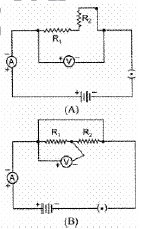a. circuit A only
b. circuit B only
c. neither of the two circuits
d. both circuits A and B
Explanation: In circuit B, the voltmeter is connected across R1 only. So the potential across the combination is not measured.

Question. Following circuits were drawn by four students, to determine the equivalent resistance of two resistors when connected in parallel. The correct circuit is drawn by the student.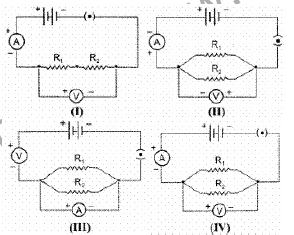a. III
b. II
c. I
d. IV
Explanation: "V" has to be connected in parallel and the potential of +ve terminal is greater than -ve terminal.

Question. A wire of resistance 2Ω is bent to form a circle. What is the resistance between two diametrically opposite points?
a. 0.5Ω
b. 4Ω
c. 1Ω
d. 1.5Ω
Explanation: When a wire of resistance 2Ω is bent to form a circle, the resistance between the two diametrically opposite points can be calculated as a parallel combination of two resistors of 1Ω each. The effective resistance of the two resistors will be 0.5Ω .

### Assertion and Reason

DIRECTION : In the following questions, a statement of assertion (A) is followed by a statement of reason (R). Mark the correct choice as:
(a) Both assertion (A) and reason (R) are true and reason (R) is the correct explanation of assertion (A).
(b) Both assertion (A) and reason (R) are true but reason (R) is not the correct explanation of assertion (A).
(c) Assertion (A) is true but reason (R) is false.
(d) Assertion (A) is false but reason (R) is true.
(e) Both Assertion and Reason are false.

Question. Assertion : Long distance power transmission is done at high voltage.
Reason : At high voltage supply power losses are less.

Question. Assertion : The electric bulbs glows immediately when switch is on.
Reason : The drift velocity of electrons in a metallic wire is very high.

Question. Assertion : The value of the current in the ammeter is the same, independent of its position in the electric circuit.
Reason : In a series combination of resistors the current is the same in every part of the circuit or the same current through each resistor.

Question. Assertion : Kirchoff’s rule follows from conservation of charge.
Reason : Kirchoff’s loop rule follows from conservation of momentum.

Question. Assertion : When a battery is short-circuited, the terminal voltage is zero.
Reason : In the situation of a short-circuit, the current is zero

Question. Assertion : Longer wires have greater resistance and the smaller wires have lesser resistance.
Reason : Resistance is inversely proportional to the length of the wire.

Question. Assertion : Silver is not used to make electric wires.
Reason : Silver is a bad conductor.

Question. Assertion : Alloys are commonly used in electrical heating devices, like electrical iron, toasters etc.
Reason : Alloys do not oxidise (burn) readily at high temperatures.

Question. Assertion : The equation V = Ri does not apply to those conducting. devices which do not obey Ohm’s law.
Reason : V = RI is a statement of Ohm’s law.

Question. Assertion : Resistivity of material may-change with temperature.
Reason : Resistivity is a material property & independent on temperature.

Question. Assertion : When the resistances are connected endto- end consecutively, they are said to be in series.
Reason : In case the total resistance is to be increased, then the individual resistances are connected in series.

Question. Assertion : Copper is used to make electric wires.
Reason : Copper has very low electrical resistance.

Question. Assertion : A resistor of resistance R is connected to an ideal battery. If the value of R is decreased, the
power dissipated in the circuit will increase.
Reason : The power dissipated in the circuit is directly proportional to the resistance of the circuit.

Question. How does the mass of wire changes when the current is flowing in the wire ?
Answer : It remains the same.

Question. How is a voltmeter connected in the circuit to measure potential difference between two points?
Answer : Voltmeter is always connected in parallel to the ends of the resistor across which the potential difference is required to be measured.

Question. Name the instrument used for measuring potential difference.
Answer :  A voltmeter is an instrument used for measuring potential difference between two points in an electric circuit.

Question. Write the formula for the heat produced (H) when a current (I) is passed through a conductor of resistance (R) for second (t).
Answer: Heat produced, H = I2Rt.

Question. Write the formula for the equivalent resistance (R) when three resistors R1, R2 and R3 are connected in (a) series, (b) parallel.
Answer: (a) R = R1 + R2 + R3
(b) 1/R = 1/R1 + 1/R2 + 1/R3

Question. Write the S.I . unit of resistivity.

Question. What happens to resistance of a conductor when its area of cross-section is increased ?

Question. How many joules are equals to 1 kWh ?

Question. What is the formula of (a) Resistance (R) of an electric appliance, (b) Safe current (I) in terms of power rating (P) and voltage rating (V).
= (Voltage rating of appliance)2/(Power rating of appliance)
R = V2/P
(b) Safe current = Power rating of appliance/Voltage rating of appliance
I = P/V

Question. Name the physical quantity that represents the ratio of potential difference and current.

Question. Define kWh.
Answer: A kilowatt hour (kWh) is the commercial unit of electrical energy. It is the energy consumed when 1 kW (1000 W) power is used for 1 hour.

Question. What are ohmic conductors ?
Answer: The conductors which obey Ohm’s law are called ohmic conductors. The V-I graph for ohmic conductor is a straight line.

Question. What are non-ohmic conductors?
Answer: The conductors which does not obey Ohm’s law are called non-ohmic conductors. The V-I graph for non-ohmic conductors is not a straight line.

Question. What will happen to the resistivity of a wire of length L if it is cut into three parts?
Answer :  Resistivity of the wire will not change even when the wire is cut into three parts as resistivity is a characteristic of the material of the conductor and does not depend on the physical dimensions of the conductor.

Question. You have two metallic wires of resistances 6 and 3 . How will you connect these wires to get an effective resistance of 2 ?
Answer :  When the equivalent resistance of connecting wire is low then, wire should be connected in parallel combination. So equivalent resistance can be obtained by the given formula :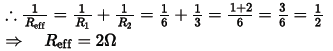Question. How will the heat produced in a resistor R change if its resistance is reduced to half of its initial value, other parameters of the circuit remain unchanged?
Answer :  The heat produced in a resistor R is given by Joule’s law of heating H = I2Rt, where I is the current and t is the time. When R becomes R/2, I will become 2I according to Ohm’s law.
Therefore, heat produced = (2I)2(R/2)t = 2H.
The heat produced will become double.

(a) Define resistance of a conductor.
(b) State Ohm’s law.
(c) State Joule’s law of heating.
Answer: (a) The obstruction offered to the flow of current by a conductor is called its resistance.
(b) According to Ohm’s law, the current flowing in a conductor is directly proportional to the potential difference applied across its ends, provided the temperature and other physical
conditions of the conductor remain constant.
(c) Joule’s law of heating states that the amount of heat produced in a conductor is directly proportional to :
1. square of current (I2),
2. resistance of wire (R), and
3. time (t), for which current is passed.

Question. The given figure shows V-I graph for two conductors A and B.
(a) Which conductor obeys Ohm’s law ?
(b) Which conductor (A or B) has more resistance? Explain your answer.
Answer: (a) Both conductors A and B obey ohm’s law.
(b) The slope of V-I graph represents resistance of a conductor.
The straight line for conductor A is steeper (or has a greater slope). Hence, conductor A has more resistance.

Question. The given figure shows three lamps and three switches 1, 2 and 3 connected with two cells.
(a) Name the switch/switches to be closed so as to light all the three lamps.
(b) How are the lamps connected : in series or in parallel ?
Answer: (a) 2 and 3, (b) in series.

Question. The atoms of copper contain electrons and the atoms of rubber also contain electrons, then, why does copper conduct electricity but rubber does not conduct electricity?
Answer: Copper contains a large number of free electrons, hence is a good conductor of electric current whereas rubber has negligible number of free electrons and is an insulator.

Question. The given figures show V-I graphs experimentally obtained for different resistors. Select the graphs for resistors that do not obey Ohm’s law.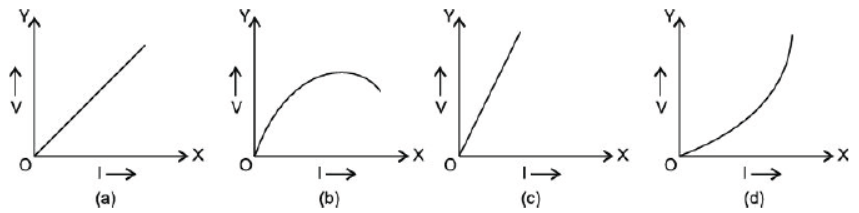Answer: The figure (b) and (d) do not obey Ohm’s law because the V-I graphs are not straight lines.

Question. An electric bulb of resistance 44 W draws a current of 5.0 A. Calculate the line voltage.
Answer: Here, I = 5.0 A, R = 44 W, V =?
Using Ohm’s law, V = IR
= 5.0 × 44 = 220 V

Question. Name and define S.I. unit of resistance.
Answer: The S.I. unit of resistance is ohm (W).
The resistance of a conductor is said to be 1 ohm if 1 ampere current flows through it when a potential difference of 1 volt is applied across the ends of the conductor.
1 ohm = 1 volt/1 ampere

Question. A given length of a wire is doubled on itself. By what factor does the resistance of the wire change ?
Answer: When given length of wire is doubled on itself, its new length L′ = L/2 and new cross-section area A′ = 2A. Hence, its new resistance
R′ = PL'/A' = P(L/2)/(2A) = 1/4 PL/A = R/4
Thus, resistance is reduced to one-fourth of its original value.

Question. Derive the relation R = R1 + R2 + R3, when resistors are joined in series.
Answer: In series combination, the same current flows in all the resistances but the potential difference across each of the resistance is different.
According to Ohm’s law, we have
V1 = IR1, V2 = IR2, V3 = IR3
If the total potential difference between A and B is V, then
V = V1 + V2 + V3
= IR1 + IR2 + IR3
= I(R1 + R2 + R3)

Question. An electric iron of resistance 20 W takes a current of 5 A. Calculate the heat developed in 30 s.
Answer: Here, Current (I) = 5 A, Resistance (R) = 20 W,
Time (t) = 30 s
∴ Heat produced (H) = I2Rt
= (5)2 × 20 × 30
= 15000 J
Thus, heat developed is 1.5 × 104 J.

Question.  On what factors does the resistance of a conductor depend?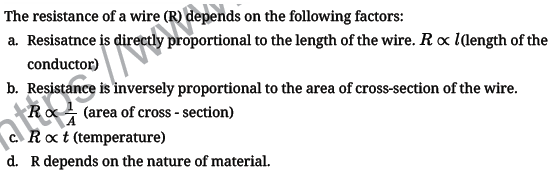Question.  The values of the current I flowing in a given resistor for corresponding values of potential difference V across the resistor are given below:
I (amperes) 0.5   1.0    2.0    3.0     4.0
V (volts)      1.6   3.4   6.7    10.2    13.2
Plot a graph between V and I and calculate the resistance of that resistor.
Answer :  Graph between I and V is as shown in figure. Since graph is almost a straight line, the slope of line between any two points give the resistance. Slope of say OB is:Question. (A) What is the resistance of a conductor?
(B) What happens to the electrical resistance when mercury is cooled to 4.12 k?
(C) What name is given to this phenomenon?
Answer :  (A) The property of a conductor due to which it opposes the flow of current through it is called resistance. The resistance of a conductor is numerically equal to the ratio of potential difference across its ends to the current flowing through it.
(B) When mercury is cooled to 4.12 K, the electrical resistance of mercury disappears completely and becomes zero and the mercury becomes super conductor.
(C) This phenomenon of loss of electrical resistance of a substance on cooling it to an extremely low temperature is known as superconductivity.

Question. A hot plate of an electric oven connected to a 220V line has two resistance coils A and B, each of 24 Ω resistance, which may be used separately, in series or in parallel. what are currents in three cases?
Answer :  Each has a resistance 24 Ω when used separately.
Isp =  220/24 = 9.17A when used separately.
when used in series Rs = 24 + 24 = 48
Isr = 220/48 = 4.58A when used in series.
when used in parallel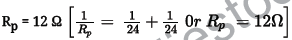Ipr = 220/12 = 18.33A when used in prallel.

Question. An electric bulb is rated 220V and 100 Ω. when operated on 110V, the power consumed will be
1. 100Ω ;
2. 75Ω
3. 50Ω ;
4. 25Ω.
P = V2/R  or R = V2/P
R = (220)2 /100
= 484Ω
Resistance of bulb whenV' =110 volts
P' =  (V')2/R  = (110)2/484 = 12100/484  = 25W

Question. A copper wire has diameter 0.5 mm and resistivity of 1.6 x 10-8 Ωm . What will be the length of this wire to make its resistance 10 Q? How much does the resistance change if the diameter is doubled?Thus, on doubling the diameter, the area of cross-section becomes 4 times and the resistance becomes one-fourth. Hence, the new resistance is 2.5Ω

Question. The given figure shows a battery, a switch and two bulbs. Complete the diagram to show the electric connections of the bulbs to the battery. How have you joined the bulbs ? Give a reason.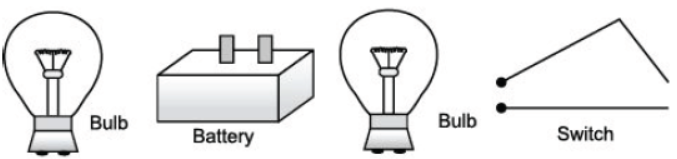Answer: The two bulbs are connected in parallel and the complete circuit diagram is drawn below :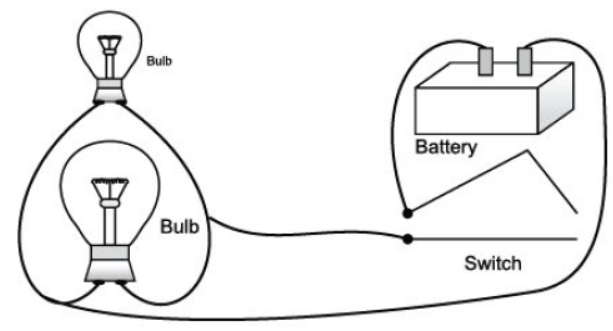The reason for connecting the two bulbs in parallel is that (i) both the bulbs glow at the same voltage, and (ii) if one bulb stops glowing, the other bulb remains unaffected.

Question. In the circuit diagram shown below, calculate : (a) total resistance and (b) current shown by the ammeter A.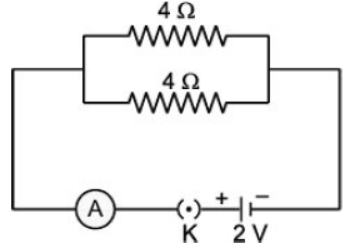Answer: (a) The two resistors of resistance 4 W each are connected in parallel.
∴ 1/R = 1/4 + 1/4
= 1 + 1/4
= 2/4 = 1/2
∴ R = 2 W
Now, Potential difference,
V = 2 V
Total resistance, R = 2 W
(b) Using Ohm’s law, Current,
I = V/R = 2/2 A= 1 A.

(a) Define electric power.
(b) What is a super conductor? Give two examples.
(c) What does an electric circuit mean?
(d) Define conductors and insulators.
Answer: (a) The electrical work done per unit time is called electric power.
(b) A superconductor is a substance of zero resistance at very low temperatures.
Example : Mercury below 4.2 K, Lead below 7.25 K.
(c) A continuous conducting path consisting of wires and other electrical components (like resistance or electric bulb, switch etc.) between the two terminals of a cell or battery, along
which an electric current flows, is called an electric circuit.
(d) The substances through which electricity can flow are called conductors and the substances through which electricity cannot flow are called insulators.

Question. A wire has a resistance of 5 W. Calculate the resistance of a wire of same material, whose length is three times and area of crosssection is four times the first wire.
Answer: Case I : R = 5 W
Let the area of cross-section be ‘A’ and length be ‘l’.
We know that, R = r. 1/A
Where r is the specific resistance of the wire.
∴ 5 = r 1/A …(i)
Case II : R1 =?
Here, length = 3l, area of cross-section = 4A
∴ R1 = r 31/4A …(ii)
Dividing equation (ii) by (i), we get
R1/5 = P.31/4A X A/P.1
∴ R1 = 5 × 3/4 = 3.75 W.

Question. While studying the dependence of potential difference (V) across a resistor on the current (I) passing through it, in order to determine the resistance of the resistor, a student took 5 readings for different values of current and plotted a graph between V and I. He got a straight line graph passing through the origin. What does the straight line signify? Write the method of determining resistance of the resistor using this graph.
Answer: The straight line in the graph signify that potential difference and current are directly proportional to each other.
The method of determining resistance of resistor using the graph is by Ohm’s law,
V = IR and by calculating the slope from the points mentioned on the graph.
∴ R = Slope of 1 - V graph

Question. An electric lamp of 100 W, a toaster of resistance 50 W, and a water filter of resistance 500 W are connected in parallel to a 220 V source. What is the resistance of an electric iron connected to the same source that takes as much current as all three appliances and what is the current through it?
Answer: The combined resistance R of the three electrical devices R1,
R2 and R3 connected in parallel is :
1/R = 1/R1 + 1/R2+ 1/R3
Here, R1 = 100 W, R2 = 50 W and R3 = 500 W
∴ 1/R = 1/100 + 1/50 + 1/500
= 5 + 10 + 1/500
= 16/500
or R = 500/6
= 31.25 W
Now, Potential difference, V = 220 V
Current, I =?
Resistance, R = 31.25 W
Using Ohm’s law : V/I = R
or 220/I = 31.25
or I = 220/7∩Δ
= 7.04 A
Hence, the current passing through the electric iron is 7.04 A.
The resistance of electric iron Riron = V/I = 220/7.04
= 31.25 W.

Question. Identify the components used in circuit diagrams represented by the following symbols :(b) A fixed resistance
(c) A variable resistance
(d) Plug key (open)
(e) Plug key (closed)
(f) A battery or combination of cells
(g) Ammeter
(h) Voltmeter

Question. Calculate the value of x if the equivalent resistance between the points P and Q as shown in figure is 5 W.Answer: Equivalent resistance of 6 W and 4 W in series,
R1 = (4 + 6) W = 10 W
Equivalent resistance of 8 W and x W in series,
R2 = (8 + x) W.
Now, R1 and R2 are in parallel.
Therefore, the equivalent resistance of R1 and R2 connected in parallel can be calculated as
1/R = 1/R1 + 1/R2
or 1/5 = 1/10 + 1/8 + x [·.· R = 5 W]
or 1/5 - 1/10 = 1/8 + x
or 2 - 1/10 = 1/8 + x
or 1/10 = 1/8 + x
or 8 + x = 10
⇒ x = 10 – 8 = 2 W
∴ Value of x = 2 W.

Question. You have three resistors of r ohm each and a battery of E volts.
How would you connect these resistors with the battery to obtain maximum current? Draw a circuit diagram to illustrate your answer and also calculate the current drawn from the battery.
Answer: Three resistors are connected in parallel. Let its equivalent resistance be R W.
∴ 1/R = 1/r + 1/r+ 1/r
1/R = 1 + 1+ 1 /r = 3/r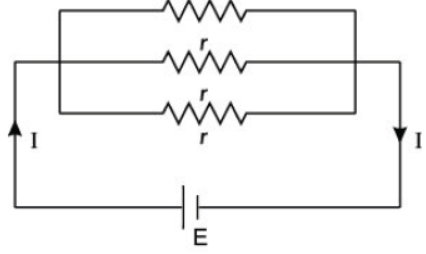⇒ R = r/3
By Ohm’s law, E = IR
⇒ Current (I) = E x 3/r = 3E/r
These resistances should be connected in parallel with the battery to obtain the maximum current.

Question. Find the current drawn from the battery by the network of four resistors shown in the figure.Answer: The given circuit can be redrawn as shown below :
Resultant resistance of R1, R2 and R3 :
R’ = R1 + R2 + R3
= 10 + 10 + 10 = 30 W
Resultant resistance of the circuit :
1/R = 1/R'+ 1/R4
= 1/30 + 1/10 = 1 + 3/30 = 4/30
R = 30/4 = 15/2 = 7.5 W
Here, V = 3 volt, I =?
By Ohm’s law, V = IR
⇒ I = V/R = 3/7.5 = 30/75 = 0.4 A

Question. Current I flowing through a resistor results in dissipation of power P. By what percentage will the power dissipated in the resistor increase, if the current through the resistor is increased by 50 percent? Justify your answer with the help of mathematical calculations.
Answer :  The power dissipated in the resistor will increase by 125 percent.
Justification using mathematical calcuations.Question. Define resistivity of material.

Question. What is the power of torch bulb rated at 2.5V and 500mA?

Question. Why series arrangement not used for connecting domestic electrical appliances in a circuit?

Question. Which has higher resistance – a 50W bulb or a 2.5W bulb and how many times?

Question. What is the direction of flow of conventional current?

Question. Why is it not advisable to handle electrical appliances with wet hands?

Question. Two electric bulbs marked 100W 220V and 200W 200V have tungsten filament of same length. Which of the two bulbs will have thicker filament?

Question. How does the resistance of a wire vary with its area of cross section?

Question. Draw the following symbols

i) Battery ii) Switch closed

iii) Resistor of resistance R iv) Voltmeter

Question. A geyser is rated 1500W, 250V. This geyser is connected to 250V mains. Calculate –

i) The current drawn

ii) The energy consumed in 50hrs.

iii) The cost of energy consumed at Rs. 2.20 per kWh.

Question. What is the function of an electric fuse? Name the material used for making fuse. In household circuit where is fuse connected?

Question. Write one important advantage of using alternative current. How alternating current differ from direct current?

ORAL QUESTIONS

Question. Which unit is equivalent of joule / coulomb?

Question. How does the resistance of a wire depend on its length?

Question. How does the resistance of a wire depend on its area of cross – section?

Question. When are resistors said to be connected in series?

Question. When are resistors said to be connected in parallel?

Question. Why is tungsten suitable for making the filament of a bulb?

Question. Why is tungsten not used as a fuse wire?

Question. Alloys are preferred over metals for making the heating elements of heaters. Why?

Question. How is the direction of electric current related to the direction of flow of electrons in a wire?

Question. Should the heating element of an electric iron be made of iron, silver or nichrome wire?

QUIZ – WHO AM I

1. I am equal to the charge carried by 6.25 x 1018 electrons.

2. I am the rate of flow of charge through any section of a conductor.

3. I am same as coulomb/second.

4. I am closed path along which electric charges can flow.

5. I am equal to the work done per unit charge from point to another.

6. I am same as joule/coulomb.

7. I oppose the flow of charges through any conductor.

8. I am same as volt/ampere.

9. I relate potential difference with current for a given resistance.

10. I am used to measure potential difference between two points of a circuit.

 CBSE Class 10 Science Chemical Reactions and Equations Assignment
 CBSE Class 10 Science Acids Bases and Salts Assignment Set A CBSE Class 10 Science Acids Bases and Salts Assignment Set B CBSE Class 10 Science Acids Bases and Salts Assignment Set C
 CBSE Class 10 Science Metals and Non Metals Assignment Set A CBSE Class 10 Science Metals and Non Metals Assignment Set B
 CBSE Class 10 Science Carbon and its compounds Assignment Set A CBSE Class 10 Science Carbon and its compounds Assignment Set B CBSE Class 10 Science Carbon and its compounds Assignment Set C CBSE Class 10 Science Carbon and its compounds Assignment Set D CBSE Class 10 Science Carbon and its compounds Assignment Set E
 CBSE Class 10 Science Periodic Classification Of Elements Assignment Set A CBSE Class 10 Science Periodic Classification Of Elements Assignment Set B CBSE Class 10 Science Periodic Classification Of Elements Assignment Set C
 CBSE Class 10 Science Biology Life Process Respiration Assignment CBSE Class 10 Science Biology Nutrition Assignment CBSE Class 10 Science Biology Respiration Assignment CBSE Class 10 Science Biology Transport Enrichment Assignment CBSE Class 10 Science Biology Transportation Assignment CBSE Class 10 Science Life Processes Assignment Set A CBSE Class 10 Science Life Processes Assignment Set B CBSE Class 10 Science Life Processes Assignment Set C
 CBSE Class 10 Science Control and Coordination Assignment Set A CBSE Class 10 Science Control and Coordination Assignment Set B CBSE Class 10 Science Control and Coordination Assignment Set C CBSE Class 10 Science Control and Coordination Assignment Set D
 CBSE Class 10 Science How do Organisms Reproduce Assignment Set A CBSE Class 10 Science How do Organisms Reproduce Assignment Set B
 CBSE Class 10 Science Heredity and Evolution Assignment Set A CBSE Class 10 Science Heredity and Evolution Assignment Set B CBSE Class 10 Science Heredity and Evolution Assignment Set C
 CBSE Class 10 Science Light Reflection and Refraction Assignment Set A CBSE Class 10 Science Light Reflection and Refraction Assignment Set B CBSE Class 10 Science Light Reflection and Refraction Assignment Set C CBSE Class 10 Science Light Reflection and Refraction Assignment Set D CBSE Class 10 Science Light Reflection and Refraction Assignment Set E CBSE Class 10 Science Light Reflection and Refraction Assignment Set F
 CBSE Class 10 Science Human Eye and Colorful World Assignment Set A CBSE Class 10 Science Human Eye and Colorful World Assignment Set B CBSE Class 10 Science Human Eye and Colorful World Assignment Set C
 CBSE Class 10 Physics Electricity Assignment Set A CBSE Class 10 Physics Electricity Assignment Set B CBSE Class 10 Physics Electricity Assignment Set C CBSE Class 10 Science Electricity Assignment Set A CBSE Class 10 Science Electricity Assignment Set B CBSE Class 10 Science Electricity Assignment Set C
 CBSE Class 10 Science Magnetic Effects of Electric Current Assignment Set A CBSE Class 10 Science Magnetic Effects of Electric Current Assignment Set B CBSE Class 10 Science Magnetic Effects of Electric Current Assignment Set C CBSE Class 10 Science Magnetic Effects of Electric Current Assignment Set D
 CBSE Class 10 Science Sources of Energy Assignment Set A CBSE Class 10 Science Sources of Energy Assignment Set B CBSE Class 10 Science Sources of Energy Assignment Set C CBSE Class 10 Science Sources of Energy Assignment Set D
 CBSE Class 10 Science Our Environment Assignment Set A CBSE Class 10 Science Our Environment Assignment Set B CBSE Class 10 Science Our Environment Assignment Set C
 CBSE Class 10 Science Sustainable Management of Natural Resources Assignment Set A CBSE Class 10 Science Sustainable Management of Natural Resources Assignment Set B CBSE Class 10 Science Sustainable Management of Natural Resources Assignment Set C
 CBSE Class 10 Physics Revision Assignment Set A CBSE Class 10 Physics Revision Assignment Set B CBSE Class 10 Physics Revision Assignment Set C CBSE Class 10 Physics Revision Assignment Set D CBSE Class 10 Physics Revision Assignment Set E CBSE Class 10 Physics Revision Assignment Set F CBSE Class 10 Physics Revision Assignment Set G CBSE Class 10 Physics Revision Assignment Set H
 CBSE Class 10 Science Assignment CBSE Class 10 Science Assignments Collection CBSE Class 10 Science Energy Crossword Puzzle Assignment
 CBSE Class 10 Physics Question Bank CBSE Class 10 Physics Tungsten Assignment
 CBSE Class 10 Science Chemistry Cleaning Capacity of Soap Assignment CBSE Class 10 Science Chemistry Determination of pH Assignment CBSE Class 10 Science Chemistry Different Types of Chemical Reactions Assignment CBSE Class 10 Science Chemistry Displacement Reaction and Reactivity Series Assignment CBSE Class 10 Science Chemistry Properties of Acetic Acid Assignment CBSE Class 10 Science Chemistry Saponification Preparation of Soap Assignment CBSE Class 10 Science Chemistry Study of properties of HCI Acid and NaOH Assignment

Tags